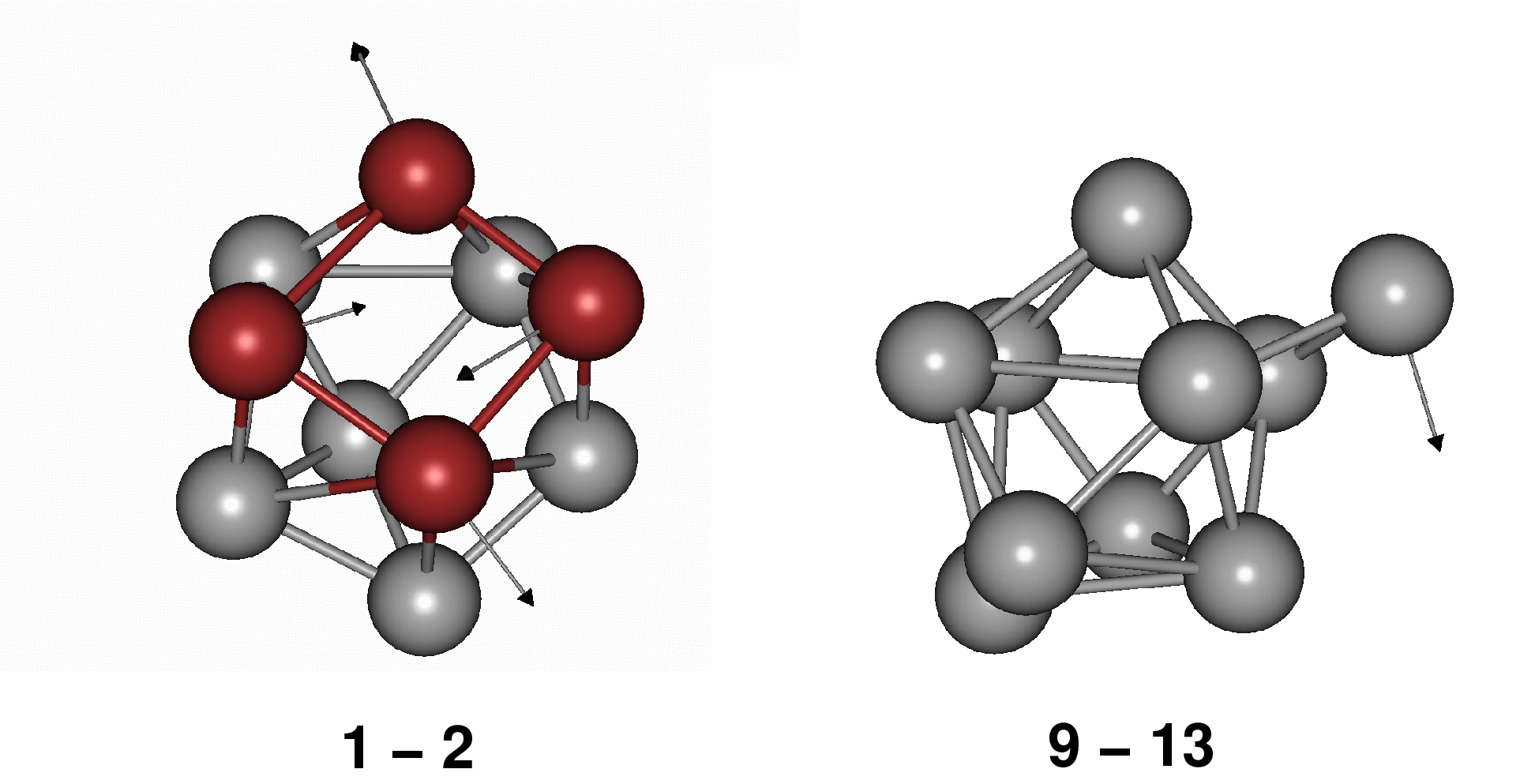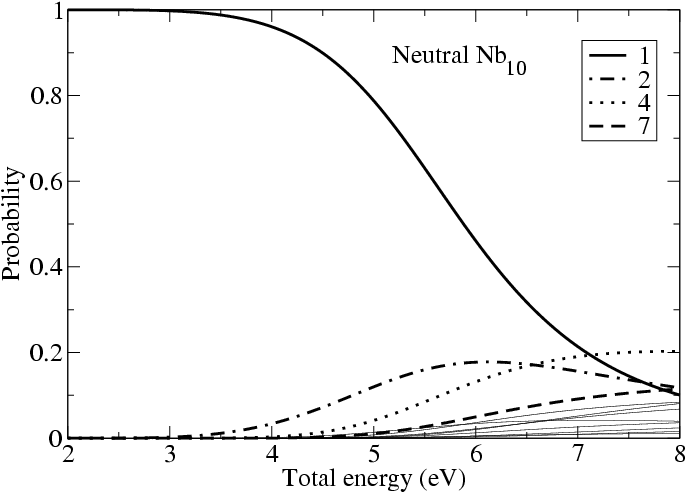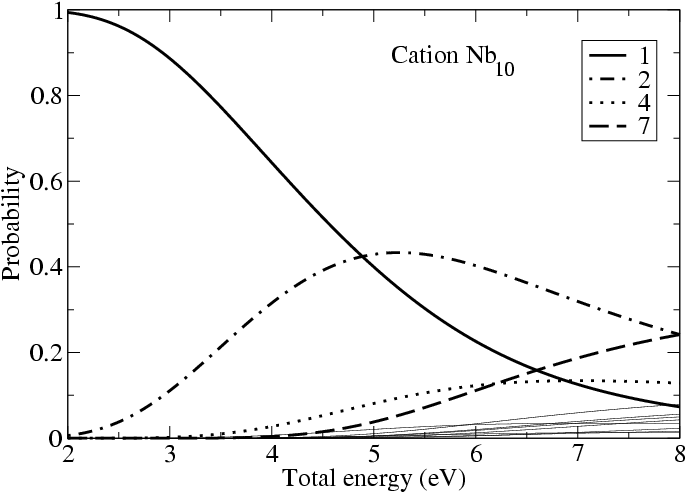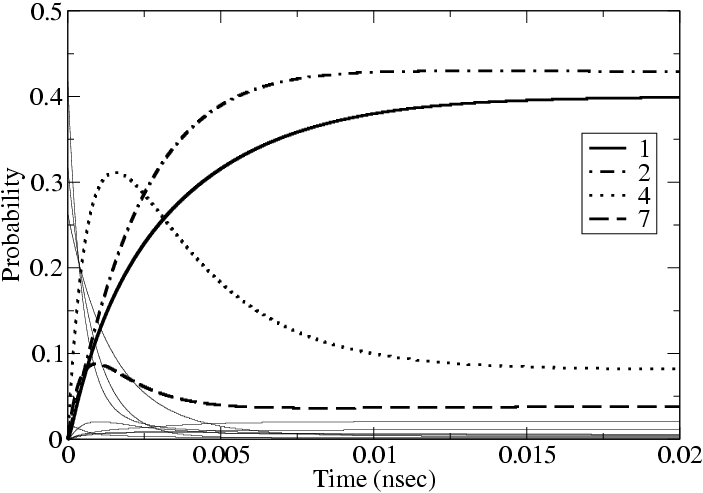# Structural Isomerism of Nb10

In this study we have applied the master equation to identify possible candidates as structural isomers in Nb10 and Nb10+. Click here to view the structures considered in this study.

Starting with the candidate global minimum structure (the bicapped square antiprism), we have mapped out rearrangements corresponding to diamond-square-diamond (DSD) and cap migration (CM) mechanisms. Example transition states (with transition vectors) are shown below for DSD (left, red atoms show the participant atoms) and CM (right).We have calculated the barriers of many possible rearrangments (out to 4 DSD arrangements away from the global minimum) using density functional theory (DFT). From these DFT data we have calculated relative rates for interconversion amongst these isomers using RRKM theory. We have used these rates and connectivities as input to the master equation (within the superposition approximation) to calculate the change in occupation of each structure as a function of time (although the time scale involved here is not necessarily realistic - instead it is the trend that matters). Within the superposition approximation, the equilibrium populations, as a function of total energy all all structures considered may also be calculated - example results are shown below for the neutral case (numbering taken from the structures figure) :However, there is much more scope for observing different structures at moderate total energies for the cation system compared with the neutral system. The equilibrium populations for the cation system are shown below:Shown below is a profile of how the populations change with time for the cation case with a total energy of 5.0 eV:From data such as these, we can make suggestions about which structures are observed as structural isomers. We conclude that for neutral Nb10, structures 1, 2, and 4 are likely candidates. For Nb10+ we have concluded that structures 1, 2, 4 and 7 are possible structural isomers. In the neutral case this has also been cross-checked against ionization potential data (measured by Knickelbein and Yang, and calculated by us). We have also calculated infrared spectra in order to guide future experimental assignments.